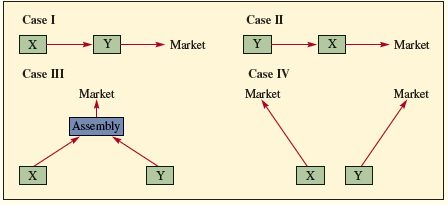# [Solved] For the Four Basic Configurations That Follow, Assume That the Market

## For the four basic configurations that follow, assume that the market is demanding product that must be processed by both Resource X and Resource Y for Cases I, II, and III.For Case IV, both resources supply separate but dependent markets; that is, the number of units of output from both X and Y must be equal.Plans are being made to produce a product that requires 40 minutes on Resource X and 30 minutes on Resource Y. Assume that there is only one of each of these resources and that market demand is 1,400 units per month.How many hours of production time would you schedule for X and Y? What would happen if both were scheduled for the same number of hours?Tags

10+ million students use Quizplus to study and prepare for their homework, quizzes and exams through 20m+ questions in 300k quizzes.

Explore our library and get Decision Science Homework Help with various study sets and a huge amount of quizzes and questions

### Related Questions

Get Free Access Now!